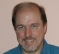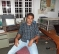# Computing the Group Delay of a Filter

I just learned a new method (new to me at least) for computing the group delay of digital filters. In the event this process turns out to be interesting to my readers, this blog describes the method. Let's start with a bit of algebra so that you'll know I'm not making all of this up.

Assume we have the N-sample h(n) impulse response of a digital filter, with n being our time-domain index, and that we represent the filter's discrete-time Fourier transform (DTFT), H(ω), in polar form as:(1)

In Eq. (1), M(ω) is the frequency magnitude response of the filter, Φ(ω) is the filter's phase response, and ω is continuous frequency measured in radians/second. Taking the derivative of H(ω) with respect to ω, we have:(2)

Dividing Eq. (2) by M(ω), we write:(3)

Performing the derivative of the first term on the right side of Eq. (3) gives us:(4)

Multiplying both sides of Eq. (4) by j/ejΦ(ω) yields:(5)

So now what does that puzzling gibberish in Eq. (5) tell us? As it turns out, it tells us a lot if we recall the following items:

• jd[H(ω)]/dω = the DTFT of n·h(n)
• M(ω)•ejΦ(ω) = H(ω) = the DTFT of h(n)
• –d[Φ(ω)]/dω = group delay of the filter.

Now we are able to translate Eq. (5) into the meaningful expression(6)

Discretizing expression (6) by replacing the DTFT with the discrete Fourier transform (DFT), we arrive at what I recently learned about computing the group delay of a digital filter:(7)

So, starting with a filter's N-sample h(n) impulse response, performing two N-point DFT's and an N-sample complex division, we can compute the filter's group delay measured in samples. (Of course, to improve our group delay granularity we can zero pad our original h(n) before computing the DFTs). The advantage of the process in expression (7) is that the phase unwrapping process needed in traditional group delay algorithms is not needed here.

WARNING Will Robinson: in implementing the process in expression (7) you must be prepared to accommodate the situation where a frequency-domain DFT[h(n)] sample is zero-valued.

POSTSCRIPT (April 9, 2016)

Recently I was using this group delay estimation method on a 6th-order IIR bandpass filter and my results appeared to be incorrect. As digital filter guru Sanjeev Sarpal pointed out to me, the above group delay estimation method works correctly for FIR filters but not always correctly for IIR filters. In fact, MATLAB's 'grpdelay()' command uses the above scheme for FIR filters, but uses a scheme called "the Shpak algorithm" for IIR filters. So, to be safe, don't use the above scheme for IIR filters.

Below is a bit of MATLAB code that can be used to demonstrate the process in expression (7).
clear, clc
Npts = 128;  % Number of plotting points

% Define filter feedforward & feedback coefficients
B = [0.03, 0.0605, 0.121, 0.0605, 0.03];
A = [1, -1.194, 0.436];

Imp_Resp_Length = 40;
[Imp_Resp,n] = impz(B,A,Imp_Resp_Length);
ImpResp_times_Time = Imp_Resp.*n;
[Freq_Resp, W] = freqz(Imp_Resp, 1, Npts, 'whole');
[Deriv_of_Freq_Resp, W] = freqz(ImpResp_times_Time, ...
1, Npts, 'whole');

Grp_Delay = real(Deriv_of_Freq_Resp./Freq_Resp);
Grp_Delay = fftshift(Grp_Delay);
Freq = (W-pi)/(2*pi);  % Freq axis vector

figure(1), clf
subplot(2,1,1)
plot(n, Imp_Resp, '-ks', ...
n, ImpResp_times_Time, '-bs', 'markersize', 4)
legend('h(n)','n times h(n)');
ylabel('Amplitude'), xlabel('n'), grid on, zoom on
subplot(2,1,2)
plot(Freq, Grp_Delay,'-rs', 'markersize', 4)
ylabel('Group Delay (samples)')
xlabel('Freq x Fs   (Fs = sample rate)')
grid on, zoom on

[ - ]
Comment by November 18, 2008For related discussion, see http://www.dsprelated.com/dspbooks/filters/Numerical_Computation_Group_Delay.html
[ - ]
Comment by November 19, 2008This is a cool trick that is also used in the built-in Matlab function grpdelay.m. The comments for grpdelay.m refer to it as: % The Smith algorithm is employed for FIR filters % - unpublished algorithm from J. O. Smith, 9-May-1988 % - faster than the Shpak algorithm, best suited to FIR filters We've been looking at group delay recently as well, for signals defined circularly (ie. we consider a repeating sequence of samples). How is group delay defined for an infinitely repeating sequence? The frequency domain representation consists of complex delta-functions - what does it mean to differentiate this? Our conclusion was that group-delay only makes sense for a finite sequence of samples.
[ - ]
Comment by November 23, 2008To Sasha Case: Humm, ... I've never considered the scenario that you describe. Group delay is a characteristic of the Fourier transform of a discrete network's impulse response. Now if you're considering an impulse response that's an infinitely-repeating sequence, wouldn't that network be more correctly called an "oscillator" rather than a "filter"? Off the top of my head I'd say that the notion of 'group delay' has no meaning when talking about oscillators. My guess is that your conclusion is correct. Regards, [-Rick-]
[ - ]
Comment by November 22, 2008To J.O. Smith: Thanks for the link to your educational 'group delay' material. As it turns out, I learned that group delay estimation method from one of the "exercises" in your Prof. Burris' book: "Computer-Based Exercises for Signal Processing Using MATLAB". (That book deserves much more recognition than it has received thus far.) By the way, I also worked (in building M4) for ESL in Sunnyvale. But I think I started at ESL after you departed. It's a good thing you weren't there the afternoon, in February 1988, when Richard Farley went on his rampage. (http://en.wikipedia.org/wiki/Richard_Farley) Regards, [-Rick Lyons-]
[ - ]
Comment by March 1, 2009Hi Rick, I have the following filter 1. CIC decimator filter ( 3 stage, decimator factor = 32, differential delay=1) with group delay of 46.5 2. Half band filter ( 10 taps ) with group delay of 5. When i cascade both filter, i get group delay of 414.5. This group delay look very high. I thought if we cascade 2 filter, then the total group delay = group delay filter A + group delay filter B Maybe this is due to the CIC decimator filter characteristic. Do you have any idea what happen here? THanks in advance for your help
[ - ]
Comment by March 2, 2009Hi cafukarfoo, Are you sure your half-band filter has 10 taps? My guess is that it's 11 taps (10 unit delay elements). Yes, when you cascade two linear time-invariant (LTI) filters, the composite group delay is the sum of the filters' individual group delays. However, the process of decimation is *not* an LTI process, so that "sum of the filters' individual group delays" concept does not apply. cafukarfoo, please accept my uncertainty here, but I'm not sure the notion of 'group delay' has any meaning when applied to a decimation process. Regards, [-Rick-]
[ - ]
Comment by April 7, 2009Hi All: In a convolution operation, how do you think about group delays? My feeling is that the end and start segments are getting distorted in convolution output. Is the group delay definition and computation same as in FIR filter? Regards, Srikanth
[ - ]
Comment by August 12, 2012Hi all, I have a question please? I have an input signal with 1.5 Mhz sampling rate. I have a serial of FIR filters which are used to interpolate this signal. The first filter interpolate the signal by 2 which works on 3 Mhz, the second one interpolate the signal by 1.5 which work on 4.5 Mhz. Could you tell me please how can I calculate the group delay of the overall system. I have an idea so that I can calculate the overall transfer function of the system than calculate the group delay of the overall system. But I am not sure if that way is right because I have two filter which they running with different sampling frequency? does any one have another idea? do you think the calculation which I do to calculate the group delay is right? Thank in advance, RDDSP
[ - ]
Comment by March 21, 2014I have 42 FIR filter coeff. I have computed group delay using above mentioned Lyon's method&found grp dly as 20.125 (in Samples). Now I found correlation between input&output of the filter, correlation bw i/p&o/p giving peak at 17th position.I m thinking, If we pass a signal through a filter, the o/p has to appear after group delay samples later, if it true grp dly and correlation value should be same. But in my case as shown above they are not same. please clear my confusion here. If I able to identify the correct delay in samples then only I can do cancellation. Because of filter coeff giving one grp dly and the correlation bw I/P&O/P showing other number, I m unable to compensate delay in samples. please help me
[ - ]
Comment by March 22, 2014Hello kovelapudi,
Please forgive my ignorance. What does "bw i/p&o/p" mean?
For your correlation processing, did you use Matlab software?

[-Rick-]
[ - ]
Comment by March 23, 2014Thank you for your reply sir. sorry for short cuts .i/p means input data stream to the filter and o/p means output data stream. I used Matlab software only for simulations. I have filtered my input data using "filter(h,1,input_data)" command. To say in complete form "output_data = filter(h,1,input_data)"; here h is 42 coefficients (42 Tap FIR) and as it is FIR i set denominator to 1 in "filter" command. Now for group delay I applied your method on this coefficients. And for correlation "xcorr" command used. say :"Y=xcorr(output_data,input_data);" Now I am expecting the position at which Y is maximum and computed group delay to be same as they both represent the number of samples delay between input and output of the filter data. Thank you
[ - ]
Comment by March 25, 2014Hello kovelapudi,
What you are doing seems sensible to me. If your filter has symmetrical real-valued coefficients, and if the filter's non-zero-valued impulse response is 42 samples in length, then the group delay should be 20.5 (measured in samples). If you send me an e-mail containing a copy of your Matlab code I'll be happy to have a look at it. My e-mail address is . I hope you can see which letters
to delete in the above e-mail address.
[-Rick-]
[ - ]
Comment by March 23, 2014Hello sir,
Can we achieve constant group delay for a "least mean squared adaptive FIR filter"?
[ - ]
Comment by March 25, 2014Hello kovelapudi,
I am shamefully ignorant of adaptive filters, so there's not much I can say about them. But if your adaptive FIR filter has symmetrical real-valued coefficients then its group delay should be constant over all frequencies (that is, a linear-phase filter).
[-Rick-]
[ - ]
Comment by March 25, 2014Hello Sir,
Thank you..
[ - ]
Comment by March 25, 2014Hello kovelapudi,
I entered my e-mail address in my first reply to you but some web page software deleted it. My e-mail address is: R.Lyons@_delete_ieee.org. I hope you can see which letters
to delete in the above e-mail address.
[-Rick-]
[ - ]
Comment by April 15, 2014Sir,
How did u define feedback and feedforward coefficients A and B in the above code.

To post reply to a comment, click on the 'reply' button attached to each comment. To post a new comment (not a reply to a comment) check out the 'Write a Comment' tab at the top of the comments.